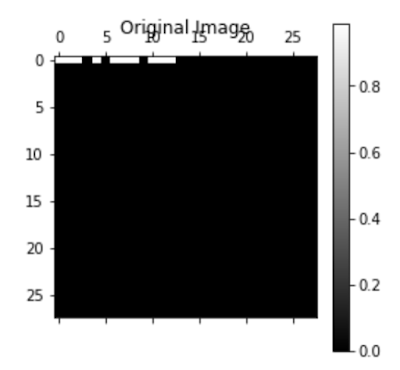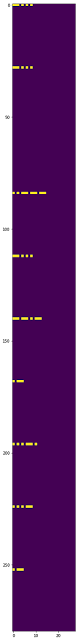## Saturday, November 25, 2017

### Introduction

Denoising auto-encoder (DAE) is an artificial neural network used for unsupervised learning of efficient codings.  DAE takes a partially corrupted input whilst training to recover the original undistorted input.

For ham radio amateurs there are many potential use cases for de-noising auto-encoders.  In this blogpost I share an experiment where I trained a neural network to decode morse code from very noisy signal.

Can you see the Morse character in the figure 1. below?   This looks like a bad waterfall display with a lot of background noise.Fig 1.  Noisy Input Image
To my big surprise this trained DAE was able to decode letter 'Y'  on the top row of the image.  The reconstructed image is shown below in Figure 2.  To put this in perspective,  how often can you totally eliminate the noise just by turning a knob in your radio?  This reconstruction is very clear with a small exception that timing of last  'dah' in letter 'Y' is a bit shorter than in the original training image.Fig 2.  Reconstructed Out Image

For reference, below is original image of letter 'Y'  that was used in the training phase.Fig 3.   Original image used for training

### Experiment Details

As a starting point I used Tensorflow tutorials using Jupyter Notebooks, in particular this excellent de-noising autoencoder example that uses MNIST database as the data source.  The MNIST database (Modified National Institute of Standards and Technology database) is a large database of handwritten digits that is commonly used for training various image processing systems. The database is also widely used for training and testing in the field of machine learning. The MNIST database contains 60,000 training images and 10,000 testing images. Half of the training set and half of the test set were taken from NIST's training dataset, while the other half of the training set and the other half of the test set were taken from NIST's testing dataset.Fig 4. Morse images
I created a simple Python script that generates a Morse code dataset in MNIST format using a text file as the input data. To keep things simple I kept the MNIST image size (28 x 28 pixels) and just 'painted' morse code as white pixels on the canvas.  These images look a bit like waterfall display in modern SDR receivers or software like CW skimmer.  I created all together 55,000 training images,  5000 validation images and 10,000 testing images.

To validate that these images look OK  I plotted first ten characters "BB 2BQA}VA" from the random text file I used for training.  Each image is 28x28 pixels in size so even the longest Morse character will easily fit on this image.  Right now all Morse characters start from top left corner but it would be easy to generate more randomness in the starting point and even length  (or speed) of these characters.

In fact the original MNIST  images have a lot of variability in the handwritten digits and some are difficult even for humans to classify correctly.  In MNIST case you have only ten classes to choose from  (numbers 0,1,2,3,4,5,6,7,8,9) but in Morse code I had 60 classes as I wanted to include also special characters in the training material.Fig 5. MNIST images

Figure 4. shows the Morse example images and Figure 5. shows the MNIST example handwritten images.

When training DAE network I added modest amount of gaussian noise to these training images.  See example on figure 6.  It is quite surprising that the DAE network is still able to decode correct answers with three times more noise added on the test images.Fig 6. Noise added to training input image

### Network model and functions

A typical feature in auto-encoders is to have hidden layers that have less features than the input or output layers.  The network is forced to learn a ”compressed” representation of the input. If the input were completely random then this compression task would be very difficult. But if there is structure in the data, for example, if some of the input features are correlated, then this algorithm will be able to discover some of those correlations.

# Network Parameters
n_input    = 784 # MNIST data input (img shape: 28*28)
n_hidden_1 = 256 # 1st layer num features
n_hidden_2 = 256 # 2nd layer num features
n_output   = 784 #
with tf.device(device2use):
# tf Graph input
x = tf.placeholder("float", [None, n_input])
y = tf.placeholder("float", [None, n_output])
dropout_keep_prob = tf.placeholder("float")
# Store layers weight & bias
weights = {
'h1': tf.Variable(tf.random_normal([n_input, n_hidden_1])),
'h2': tf.Variable(tf.random_normal([n_hidden_1, n_hidden_2])),
'out': tf.Variable(tf.random_normal([n_hidden_2, n_output]))
}
biases = {
'b1': tf.Variable(tf.random_normal([n_hidden_1])),
'b2': tf.Variable(tf.random_normal([n_hidden_2])),
'out': tf.Variable(tf.random_normal([n_output]))
}

The functions for this neural network are below. The cost function calculates the mean square of the difference of output and training images.

with tf.device(device2use):
# MODEL
out = denoising_autoencoder(x, weights, biases, dropout_keep_prob)
# DEFINE LOSS AND OPTIMIZER
cost = tf.reduce_mean(tf.pow(out-y, 2))

# INITIALIZE
init = tf.initialize_all_variables()
# SAVER
savedir = "nets/"
saver = tf.train.Saver(max_to_keep=3)

### Model Training

I used the following parameters for training the model. Training took  1780 seconds on a Macbook Pro laptop. The cost curve of training process is shown in Figure 6.

training_epochs = 300
batch_size      = 1000
display_step    = 5
plot_step       = 10Fig 6. Cost curve
 It is interesting to observe what is happening to the weights.  Figure 7 shows the first hidden layer "h1" weights after training is completed. Each of these blocks have learned some internal representation of the Morse characters. You can also see the noise that was present in the training data.Fig 7.  Filter shape for "h1" weights

### Software

The Jupyter Notebook source code of this experiment has been posted to Github.  Many thanks to the original contributors of this and other Tensorflow tutorials. Without them this experiment would not have been possible.

### Conclusions

This experiment demonstrates that de-noising auto-encoders could have many potential use cases for ham radio experiments. While I used MNIST format (28x28 pixel images) in this experiment, it is quite feasible to use other kinds of data, such as audio WAV files,  SSTV images  or some data from other digital modes commonly used by ham radio amateurs.

If your data has a clear structure that will have noise added and distorted during a radio transmission, it would be quite feasible to experiment implementing a de-noising auto-encoder to restore  near original quality.   It is just a matter of re-configuring the DAE network and re-training the neural network.

If this article sparked your interest in de-noising auto-encoders please let me know.  Machine Learning algorithms are rapidly being deployed in many data intensive applications.  I think it is time for ham radio amateurs to start experimenting with this technology as well.

73
Mauri  AG1LE